# High School Chemistry : Chemical Reactions

## Example Questions

← Previous 1 3 4 5 6 7

### Example Question #1 : Help With Equilibrium Constant

Consider the following balanced reaction: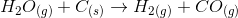What is the equilibrium expression for this reaction?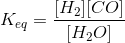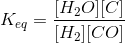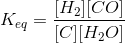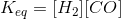Explanation:

When writing the equilibrium expression for a reaction, remember that the products are on the top of the expression and the reactants are on the bottom. Any coefficients in the balanced reaction become the exponents in the equilibrium constant.

A general formula and corresponding equilibrium expression are given here: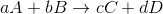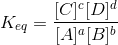Our equation follows the same format. Note, however, that pure solids and liquids are not included in the equilibrium expression, but pure gases (such as hydrogen) are. This is because the pressure of pure gases can affect equilibrium, but manipulating pure solids or liquids cannot.### Example Question #2 : Help With Equilibrium Constant

Consider the following reaction: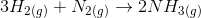What is the equilibrium constant for this reaction?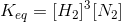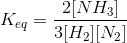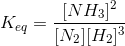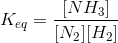Explanation:

When writing the equilibrium expression for a reaction, remember that the products are on the top of the expression and the reactants are on the bottom. Any coefficients in the balanced reaction become the exponents in the equilibrium constant.

A general formula and corresponding equilibrium expression are given here:Our equation has only one product, but follows the same format.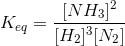Note that pure solids and liquids are not included in the equilibrium expression, but pure gases (such as nitrogen) are. This is because the pressure of pure gases can affect equilibrium, but manipulating pure solids or liquids cannot.

### Example Question #3 : Help With Equilibrium Constant

What equation gives the equilibrium constant for the following reaction?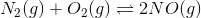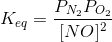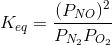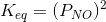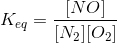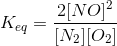Explanation:

The general formula for the equilibrium constant of a reaction is: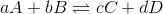For gaseous reactions, however, partial pressure can be substituted for concentration. For the given reaction, this means our equilibrium constant will be equal to the partial pressure of the products divided by the partial pressure of the reactants.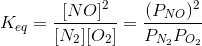### Example Question #4 : Help With Equilibrium Constant

Which of the following will affect the equilibrium constant of a reaction?

None of these will affect the reaction equilibrium constant

Product concentration

Ratio of substrate concentration to product concentration

Substrate concentration

None of these will affect the reaction equilibrium constant

Explanation:

The equilibrium constant gives the ratio of product concentration to reactant concentration that results in equal forward and reverse rates of reaction. Essentially, the equilibrium constant defines the parameters of equilibrium for a given reaction. Altering the concentration of reactants or products will change the state of the reaction, driving it to produce more reactants or products in order to accommodate the change (Le Chatelier's principle), but will not alter the equilibrium parameters. Adding an enzyme will increase the rate of reaction by decreasing the activation energy, but will still not affect the equilibrium ratios.

The main factor that is capable of altering the equilibrium constant is change in temperature, which alters the amount of energy available to the system and affects the balance of equilibrium.

### Example Question #5 : Help With Equilibrium Constant

Aluminum hydroxide is an insoluble salt. What is the solubility product expression for aluminum hydroxide?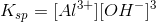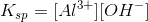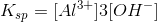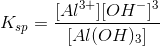Explanation:

When creating the solubility product expression for a salt, keep in mind that salts are solids, and are therefore not included in the expression. Also, the coefficients in the balanced reaction become exponents in the expression.

For a general reaction, the solubility product constant would be given as follows: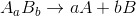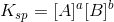Aluminum hydroxide will dissociate based on the following balanced reaction: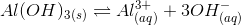As a result, the solubility product expression is:### Example Question #1 : Chemical Reactions

What is the difference between the reaction quotient and the equilibrium constant?

The equilibrium constant is valid only at equilibrium, and the reaction quotient can be calculated at any given moment in the reaction.

There is no difference between the two.

The reaction quotient  is valid only at equilibrium, and the equilibrium constant can be calculated at any given moment in the reaction.

The reaction quotient is equivalent to the rate law.

The equilibrium constant is valid only at equilibrium, and the reaction quotient can be calculated at any given moment in the reaction.

Explanation:

The correct answer gives the accurate definition of both the equilibrium constant and the reaction quotient.

### Example Question #1 : Equilibrium

What is the equilbrium constant for a reaction written in reverse if the forward reaction has constant K?

1/K

2K

4K

K/2

1/K

Explanation:

This is one of the properties of the law of mass action. The equilbrium constant for a reaction written in reverse is 1/K.

### Example Question #1 : Equilibrium

Which of the following factors will change the equilibrium constant, Keq?

Adding a chemical that will cause side reactions

Change in temperature

Change in solvent volume

Change in temperature

Explanation:

The only factor that changes the equilibrium constant is temperature. Changes in concentration of reactants or products by any means (whether addition, taking away solvent, or adding a chemical that will cause side reactions) will remove the system from equilibrium, but will not change the equilibrium constant.

### Example Question #1 : Equilibrium

For the following reaction, what would be the correct equilibrium expression?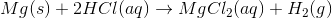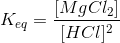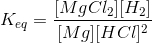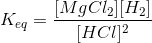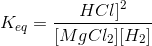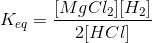Explanation:

For any equilibrium expression, solids and liquids are excluded because their concentrations are presumed to be constant during the reaction. The equilibrium expression is defined as the ratio of the concentration of products divided by the concentration of the reactants. Each reactant or product is raised to the power corresponding to its coefficient in the balanced chemical equation.

Using an arbitrary example: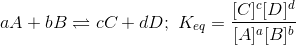Compare this to our question. Remember to exclude the solid magnesium!### Example Question #1 : Chemical Reactions

Determine the equation for the equilibrium constant of the following unbalanced reaction: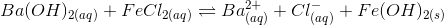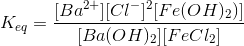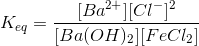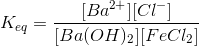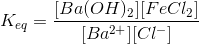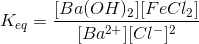Explanation:

The equilibrium constant,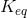, is the ratio of the concentration of products raised to their coefficients, over the concentration of reactants raised to their coefficients. To find its value, we first need to balance the equation and then consider only the products and reactants that actually have concentrations (i.e. aqueous and gaseous species). Liquids and solids can be omitted from the calculation.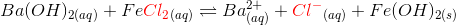We note that chlorine only appears once on the right, so we add a 2 coefficient to balance the equation: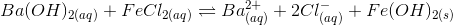Since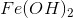is a solid (most hydroxides precipitate, unless they are paired with alkali metals, barium, or calcium) it will not be included in the equilibrium constant calculation.

The chloride ion concentration is raised to the second power because of the 2 coefficient that we added. So, putting products (species on the right side, other than the solid) on the top and reactants (species on the left) on bottom, we get:← Previous 1 3 4 5 6 7

### All High School Chemistry Resources